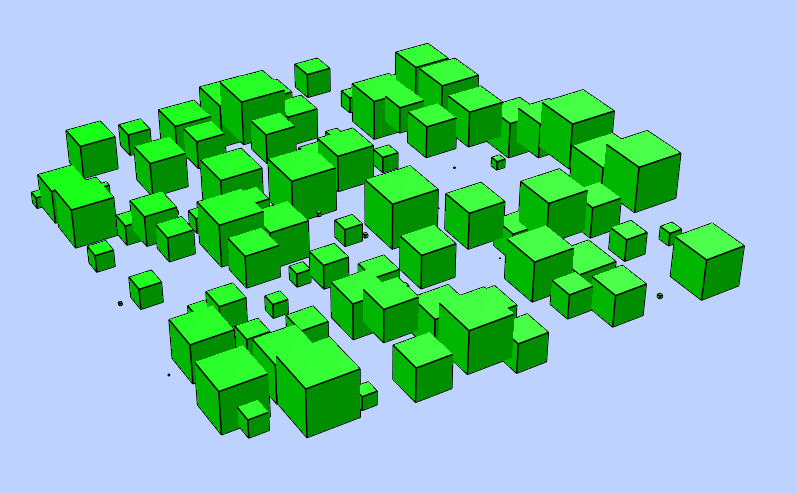# POLYFACE¶

Create a new polyface entity, polyface is a dxf-polyline entity!. A Polyface consist of one or more faces, where each face can have three or four 3D points.

`DXFEngine.``polyface`(precision=6, **kwargs)
Parameters: precision – vertex-coords will be rounded to precision places, and if the vertex is equal to an other vertex, only one vertex will be used, this reduces filespace, the coords will be rounded only for the comparison of the vertices, the output file has the full float resolution.

The flags-bit POLYLINE_POLYFACE is set.

for kwargs see POLYLINE

## Methods¶

`Polyface.add_face(self, vertices, color=0):`

This is the recommend method for adding faces.

Parameters: vertices – is a list or tuple with 3 or 4 points (x,y,z). color (int) – range [1..255], 0 = BYBLOCK, 256 = BYLAYER

## Example¶

```from dxfwrite import DXFEngine as dxf

def get_cube(basepoint, length):
def scale( point ):
return ( (basepoint+point*length),
(basepoint+point*length),
(basepoint+point*length))
pface = dxf.polyface()
# cube corner points
p1 = scale( (0,0,0) )
p2 = scale( (0,0,1) )
p3 = scale( (0,1,0) )
p4 = scale( (0,1,1) )
p5 = scale( (1,0,0) )
p6 = scale( (1,0,1) )
p7 = scale( (1,1,0) )
p8 = scale( (1,1,1) )

# define the 6 cube faces
# look into -x direction
# On dxf output double vertices will be removed.
pface.add_face([p1, p5, p7, p3], color=1) # base
pface.add_face([p1, p5, p6, p2], color=2) # left
pface.add_face([p5, p7, p8, p6], color=3) # front
pface.add_face([p7, p8, p4, p3], color=4) # right
pface.add_face([p1, p3, p4, p2], color=5) # back
pface.add_face([p2, p6, p8, p4], color=6) # top
return pface

def simple_faces():
pface = dxf.polyface()
p1 = (0,0,0)
p2 = (0,1,0)
p3 = (1,1,0)
p4 = (1,0,0)

p5 = (0,0,1)
p6 = (0,1,1)
p7 = (1,1,1)
p8 = (1,0,1)

pface.add_face([p1, p2, p3, p4]) # base
pface.add_face([p5, p6, p7, p8]) # top
```